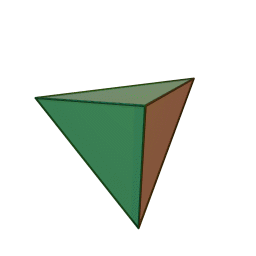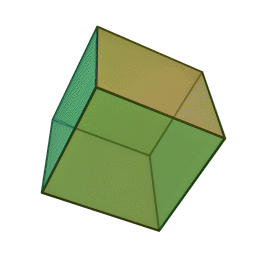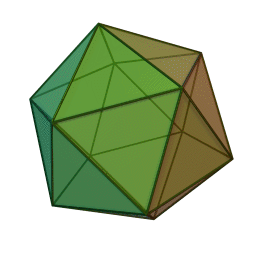# Group Theory: The Mathematics of Symmetry?

Often you’ll hear people describe group theory as being “the mathematics of symmetry.” If you’ve been exposed to a little bit of group theory, you might find this a little mystifying. While there are some subsets of group theory that explicitly pertain to symmetry (in particular, the dihedral groups, representing the symmetries of regular polygons), there are many more groups that seem to have nothing to do with symmetry (the rational numbers, for example, or the dicyclic groups). Much of group theory involves proving theorems about the structure of groups in abstract, and many of these theorems again seem to tell us little to nothing about symmetry (what does “for every prime p that divides the size of a group G, there is a subgroup of G with order p” mean as a statement about the nature of symmetry?). So what’s up? Why this identification between groups and symmetry?

Well, I think we can start with asking what we even mean by ‘symmetry’. The first thing I think of when I hear the word ‘symmetry’ is geometric symmetry – the rotations, translations, and reflections that hold fixed a given shape. This is indeed one big part of symmetry, but not all of it. Symmetry is huge in physics as well, and the meaning in this context is rarely geometric symmetry. Instead, ‘symmetry’ finds its deepest application in the notion of symmetries of nature, meaning transformations that hold fixed the form of the laws of nature. For instance, the behavior of physical systems would be identical if we were to uniformly “slide” the universe in one direction by some fixed amount. So the laws of nature must be invariant with respect to this type of uniform translation, and we say that uniform spatial translation is a symmetry of nature. But the behavior of physical systems would be affected if we, say, began uniformly accelerating all of space in some direction. So uniform acceleration is not a symmetry of nature, and the laws of nature will be sensitive to these types of transformations. In this context, symmetry is more or less synonymous with invariance.

What’s the similarity between these two senses of symmetry? Invariance really is the key. Geometric symmetries are transformations that hold fixed certain geometric properties; physical symmetries are transformations that hold fixed certain physical properties. Fundamentally, symmetries are transformations that hold fixed some quantity. When you choose strange abstract qualities, your symmetries can get pretty weird (like the invariance of magnetic fields with respect to addition of curl-less vector fields to the magnetic vector potential, one of the two gauge symmetries of Maxwell’s equations). But they are symmetries nonetheless!

Now, how does this expansive concept of symmetries relate to groups? Well, looking at the group axioms, it becomes fairly obvious that they do actually fit pretty well with the concept. Let’s think of the elements of a group as transformations one could perform upon a system, and the group operation as being ‘transformation composition’ for successive transformations. And finally, we think of the group as being a complete set of symmetries. Now we’ll look at what the group axioms say, one at a time.

1. Closure
For any two elements a, b in G, a•b is in G.
– Translation: If two transformations each individually hold fix some quantity, then doing the two transformations successively will also hold fixed the quantity.
2. Associativity
For any a, b, c in G, (a•b)•c = a•(b•c)
– Translation: The order in which transformations are applied to an object is all that determines the nature of the net transformation. The notion of parentheses doesn’t make sense in this context, there is only the left-to-right order of the application of transformations.
3. Identity
There is an element e in G such that for any a in G, a•e = e•a = a.
– Translation: Doing nothing at all holds fixed whatever quantity you choose.
4. Inverses
For any a in G, there is an element a’ in G such that a•a’ = e
– Translation: Any transformation that holds fixed some quantity will also hold fixed that quantity if done in reverse.

Put together, we see that it makes sense to think about a group as the complete set of reversible transformations that hold fixed a certain quantity. (To be clear, this indicates that any such set of symmetries can be treated as a group, not necessarily that any group can be regarded as a set of symmetries. However, this does turn out to be the case! And in fact, any group whatsoever can be considered as a set of geometric symmetries of some abstract object!)

“Complete” needs to be fleshed out a bit more. When talking about a set of transformations, we need to be picking from a well-defined starting set of ‘allowed transformations’. For example, sets of geometric symmetries of objects are usually drawn from the set of all isometries (transformations that keep all distances the same, i.e. rotations, reflections, and translations). Instead of this, we could also consider the allowed transformations to be restricted to the set of all rotations, in which case we’d just be looking at the set of all rotations that don’t change the object. But in general, we are not allowed to talk about the set of possible transformations as just being some subset of the set of all rotations. Why? Because we require that the set of allowed transformations be closed. If a certain rotation is in consideration as an allowed transformation of an object, then so must be twice that rotation, thrice it, and so on. And so must be the reverse of that rotation. Not all sets of rotations satisfy this constraint!

The general statement is that the set of allowed transformations from which we draw our set of symmetries must be closed. And if it is, then the resulting set of symmetries is well described as some group!

So there is a fairly simple sense in which group theory is the study of symmetries. There are the obvious geometric symmetries that I already mentioned (the dihedral groups). But now we can think further about what other familiar groups are actually symmetries of. (, +) is a group, so what are the integers symmetries of? There’s actually a few answers that work here. One is that is the set of translational symmetries of an infinite line of evenly spaced dots. Translating to the right corresponds to positive numbers, to the left is negatives, and no translation at all is 0. What about (n, +)? It is the set of rotational symmetries of a circle with n dots placed evenly along its perimeter. I encourage you to try to think about other examples of groups (symmetric and alternating groups, ℚ, and so on), and how to think about them as symmetry groups.

This way of thinking about groups is more than just visually appealing, it actually helps clarify some otherwise opaque results in group theory. For example, why is the stabilizer of a group action always a subgroup? Well, the group action defines a set of allowed transformations, and the stabilizer of x is exactly the set of transformations that hold x fixed! So we have an invariant quantity x, and thus it is perfectly reasonable that the stabilizer should be a group.

Thinking of groups as sets of symmetries also allows us a nice physical interpretation of the idea of conjugacy classes, a hugely important tool in group theory. Remember, the conjugacy class of an element x in G is just the set of all conjugates of x; Cl(x) = {gxg-1 for all g in G}. This ABA-1 pattern might be familiar to you if you’ve studied a little bit of matrix representations of coordinate systems in physics. In this context, ABA-1 just represents a coordinate transformation of B! First we apply some transformation A that changes our reference frame, then we apply B, and then we undo A to come back to the original reference frame. The result is basically that we get a transformation that are just like B, but in a different reference frame! So it makes perfect sense to think of the conjugacy class of an element x as just the set of all elements of the same “type” as x.

For a dihedral group, we get one conjugacy class for the identity, another for all rotations, and another for all flips. Why? Well every flip can be represented as any other flip in a rotated reference frame! And similarly, every rotation can be represented as any other rotation in a flipped reference frame. And of course, the do-nothing transformation will look the same in all reference frames, which is why the identity has its own conjugacy class. And for another example, you can see some great visualizations of the conjugacy class breakdown of the cube’s symmetry group here, under Examples at the top. In general, looking at conjugacy classes is a good way to get a sense of the natural breakdown of categories in your set of symmetries.

Now, the famous theorems about group structure (Lagrange, Cauchy, Sylow) all have intriguing interpretations as applied to symmetries. Why must it be that any object with a prime number of symmetries cannot have any non-trivial smaller complete sets of symmetries? And doesn’t it seem totally non-obvious that an object that has a complete set of symmetries whose size is divisible by 3, say, must also have a smaller complete set of symmetries (one drawn from a smaller closed set of allowed transformations) whose size is 3? But it’s true! Think about the symmetries of a regular triangle. There are six of them, and correspondingly there’s a closed set of symmetries of size 3, namely, the do-nothing transformation and the two rotations! And the same thing applies for any symmetry group of size 3N.

Furthermore, Sylow’s theorems give us really strong constraints on the number of subgroups of various sizes, none of which seem at all intuitive. Why must it be that if an object has 9N symmetries for some N that’s not divisible by 3, then N must be divisible by the number of smaller complete sets of symmetries?? Thinking about and trying to make intuitive sense of these connections is quite mind-boggling to me.

# Symmetries of Platonic Solids

Definition: A polyhedron is regular if all its faces are the same regular polygon, and all angles are equal.

It turns out that there are only five Platonic solids (convex regular polyhedra).

Here’s a quick proof:

• For a regular n-gon, the sum of its interior angles is (n – 2)π.
• So the angle at any one of its corners must be (n – 2) π/n.
• Now, suppose we have r faces (each a regular n-gon) meeting at every vertex.
• Since the meeting point for each face is a corner with angle (n – 2)π/n, we have that the sum of the angles is r (n – 2) π/n.
• And since the polyhedron is convex we must have r (n – 2) π/n < 2π.
• This can be rewritten as (r – 2)(n – 2) < 4.
• The only positive integers satisfying this are (nr) = (3, 3), (4, 3), (3, 4), (5, 3), (3, 5).
• These are our five Platonic solids!

(3,3): TetrahedronSymmetry group: S4

(4,3): CubeSymmetry group: S4 × 2

(3,4): OctahedronSymmetry group: S4 × 2

(5,3): DodecahedronSymmetry group: A5 × 2

(3,5): IcosahedronSymmetry group: A5 × 2

— — —

Here’s one more thing to notice; when looking at small dihedral groups, we saw that Dih3 (the symmetry group for a regular triangle) was isomorphic to S3 (the set of permutations of three items). We also saw that this isomorphism did not hold for higher order dihedral groups. In other words, the only regular polygon whose symmetry group was some symmetric group was the triangle.

Now we see that the tetrahedron (which we might consider to be the closest thing to a three-dimensional version of a regular triangle) has as its symmetry group S4. In other words, we get to S4 not by moving to polygons with more sides, but by moving to a higher dimension!

This may bring a conjecture to our minds: perhaps in general, moving up dimensions is the way to get regular geometric shapes that are isomorphic to higher order symmetric groups. That is, perhaps the symmetry group of a regular “triangle-like” objects in n dimensions is isomorphic to Sn. So this would predict that there is some 4D version of the 2D triangle and 3D tetrahedron whose symmetry group is S5. And guess what; this turns out to be right!

The higher dimensional generalization of a triangle and tetrahedron is called a n-simplex. And the symmetry group for each of these simplexes is in fact a symmetric group! So all symmetric groups can be considered to be descriptions of the set of symmetries of some possibly very high-dimensional tetrahedron! This is a fun little piece of trivia that I don’t know quite what to make of.